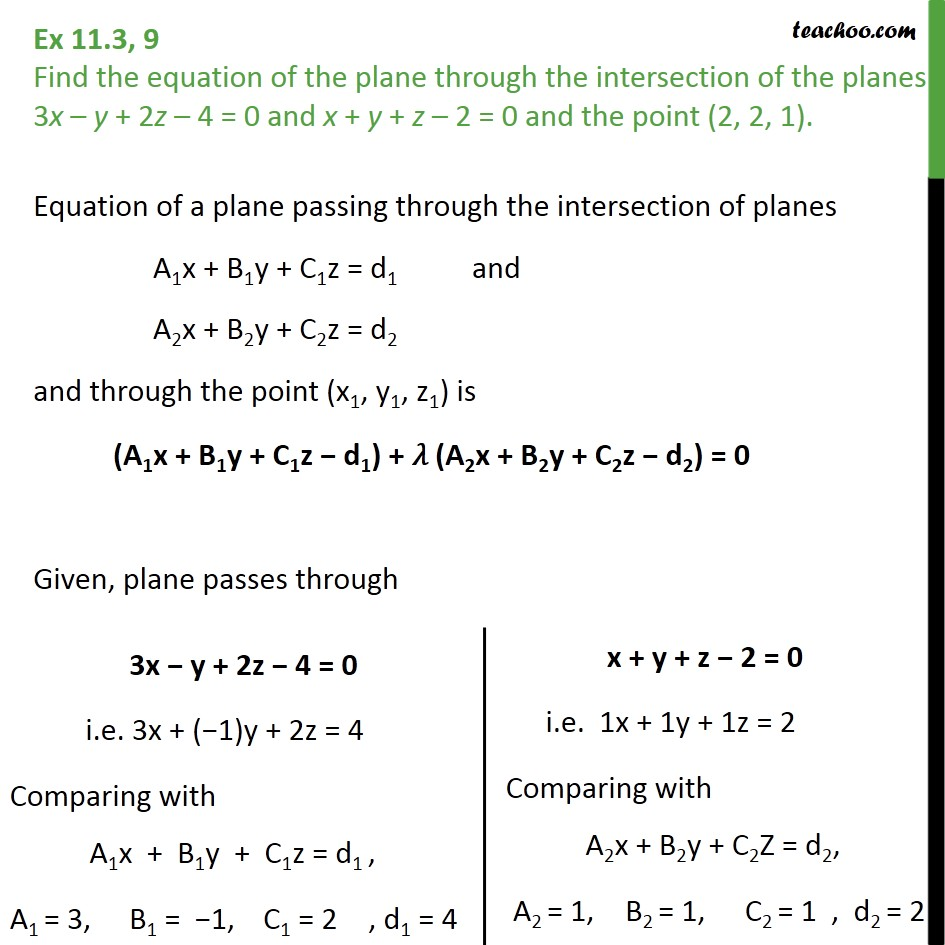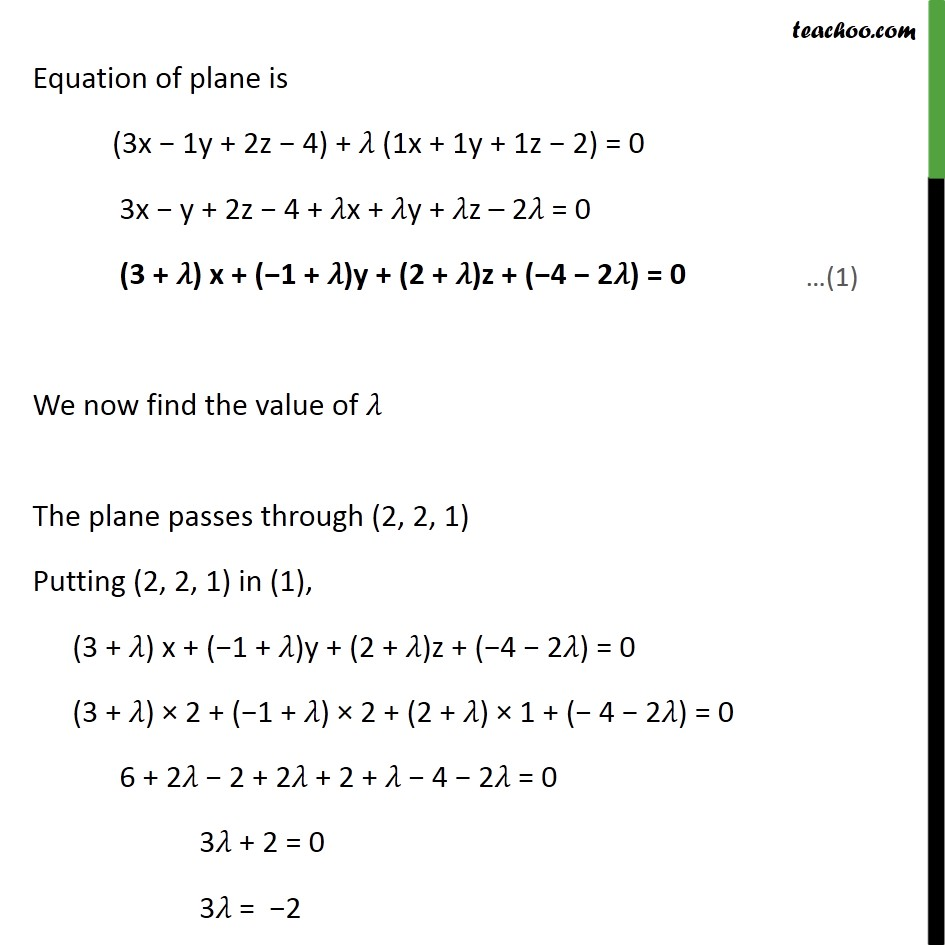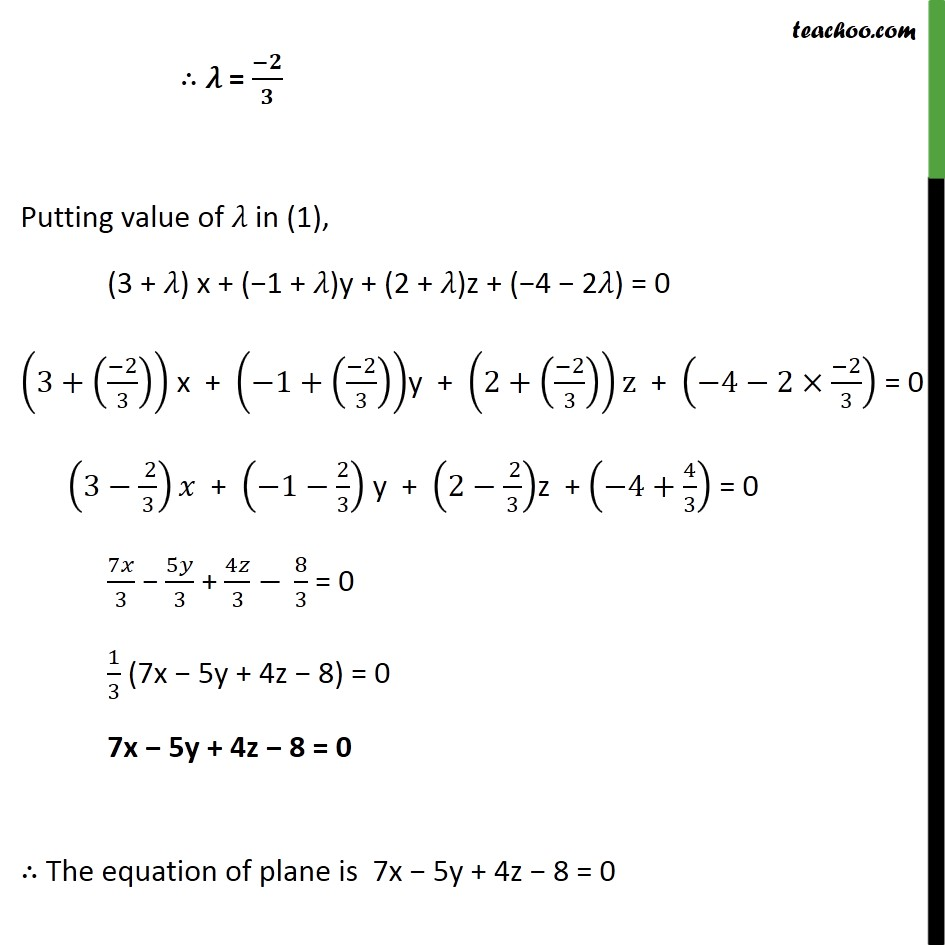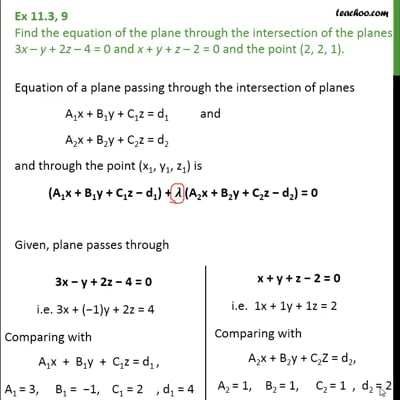Ex 11.3

Chapter 11 Class 12 Three Dimensional Geometry
Serial order wiseThis video is only available for Teachoo black users

Solve all your doubts with Teachoo Black (new monthly pack available now!)

### Transcript

Ex 11.3, 9 Find the equation of the plane through the intersection of the planes 3x y + 2z 4 = 0 and x + y + z 2 = 0 and the point (2, 2, 1). Equation of a plane passing through the intersection of planes A1x + B1y + C1z = d1 and A2x + B2y + C2z = d2 and through the point (x1, y1, z1) is (A1x + B1y + C1z d1) + (A2x + B2y + C2z d2) = 0 Given, plane passes through Equation of plane is (3x 1y + 2z 4) + (1x + 1y + 1z 2) = 0 3x y + 2z 4 + x + y + z 2 = 0 (3 + ) x + ( 1 + )y + (2 + )z + ( 4 2 ) = 0 We now find the value of The plane passes through (2, 2, 1) Putting (2, 2, 1) in (1), (3 + ) x + ( 1 + )y + (2 + )z + ( 4 2 ) = 0 (3 + ) 2 + ( 1 + ) 2 + (2 + ) 1 + ( 4 2 ) = 0 6 + 2 2 + 2 + 2 + 4 2 = 0 3 + 2 = 0 3 = 2 = Putting value of in (1), (3 + ) x + ( 1 + )y + (2 + )z + ( 4 2 ) = 0 3+ 2 3 x + 1+ 2 3 y + 2+ 2 3 z + 4 2 2 3 = 0 3 2 3 + 1 2 3 y + 2 2 3 z + 4+ 4 3 = 0 7 3 5 3 + 4 3 8 3 = 0 1 3 (7x 5y + 4z 8) = 0 7x 5y + 4z 8 = 0 The equation of plane is 7x 5y + 4z 8 = 0